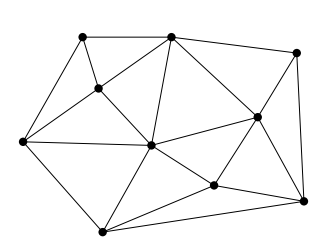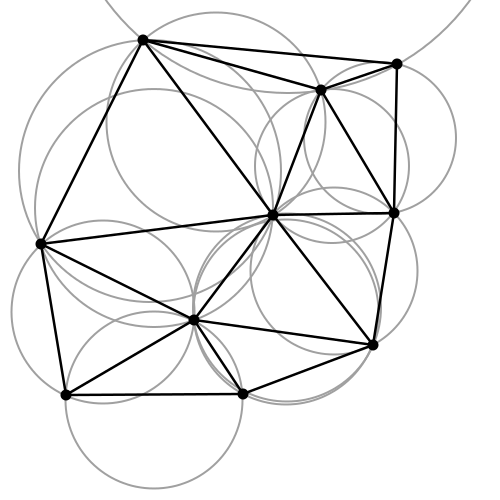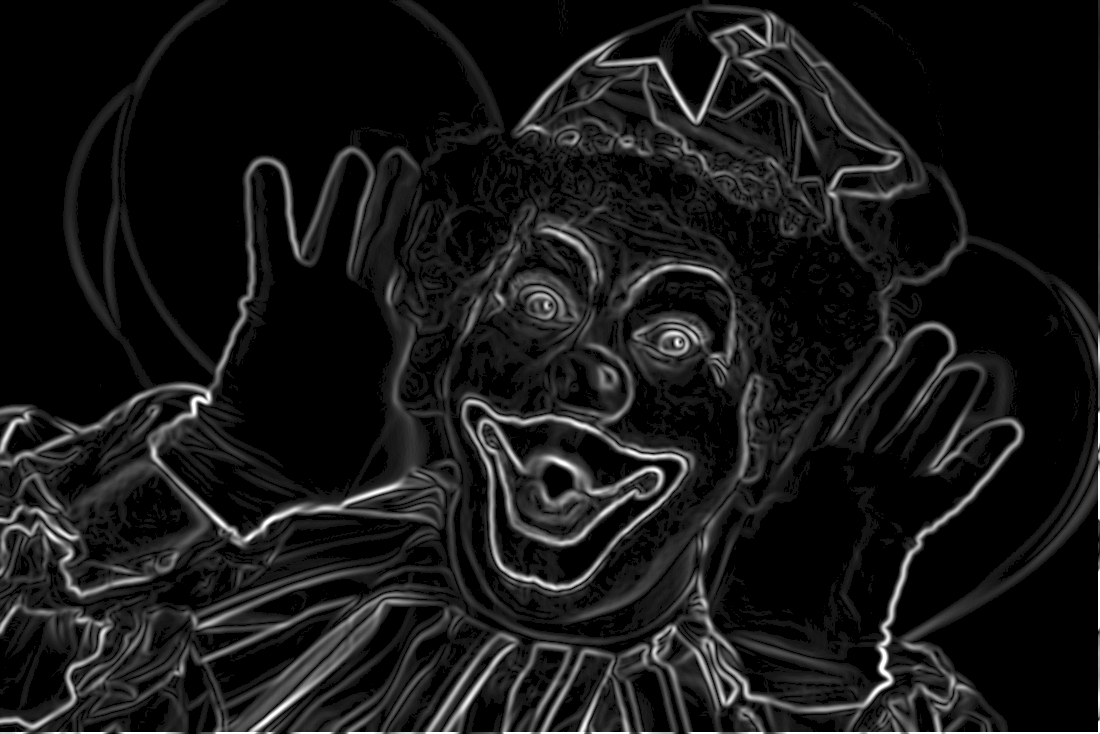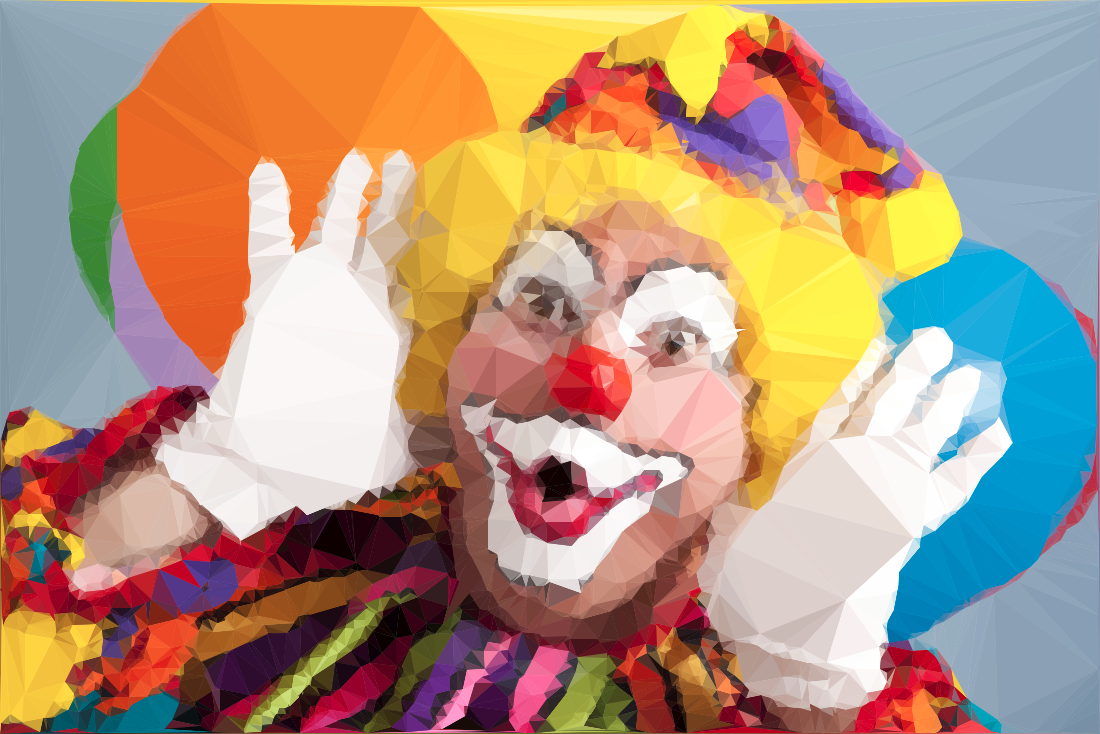Over a million developers have joined DZone.{{announcement.body}}
{{announcement.title}}

DZone's Guide to

# How Math and Computational Geometry Influence Digital Art

This is the first of a series of articles that will highlight interesting image processing techniques. It is an attempt to explore the fields of computer technology and art.

· AI Zone ·
Free Resource

Comment (0)

Save
{{ articles.views | formatCount}} Views

Adding AI to your task-oriented bots enables advanced abilities such as bringing structure to your unstructured data. Get our free platform for developers today.

In this article, we present a technique to triangulate source images, converting them to abstract, somewhat artistic images composed of tiles of triangles. This will be an attempt to explore the fields of computer technology and art.

But first, what is triangulation? Simply spoken, triangulation partitions a polygon into triangles, which allows, for instance, to compute the area of a polygon and make some operations on the computed polygon surface — for example, to interpolate the triangulated area color palette and repaint it with the obtained average color. In other terms, the triangulation might be conceived as a geometric object defined by a point set, but what differentiates the polygons from a point set is that the latter does not have an interior, except if we treat the point set as a convex hull/polygon. But to think of a point set as a convex polygon, the points from the interior of the convex hull should not be ignored completely. This is what differentiates the Delaunay triangulation from the other triangulation techniques.Figure 1: Point set triangulation.

## Delaunay Triangulation

Wikipedia has a very succinct definition of the Delaunay triangulation:

“…a Delaunay triangulation (also known as a Delone triangulation) for a given set P  of discrete points in a plane is a triangulation DT(P) such that no point in P is inside the circumcircle of any triangle in DT(P).”

What does this mean?

The circumcircle of a triangle is the unique circle passing through all the vertices of the triangle. This will get valuable meaning in the following sections. So, considering that we have a set of six points, we can obtain a triangulated polygon by tracing a circle around each vertex of the constructed triangle in such a manner that the circumcircle of all five triangles is empty. We also say that the triangles satisfy the empty circle property (Figure 2).Figure 2: All triangles satisfy the empty circle property.

After this short theoretical introduction, we want to apply this technique to a more practical yet aesthetical field, concretely to transform an input image to its triangulated counterpart. Having the basic idea and its theoretical background, we can start to construct the basic building blocks of the algorithm.

Above all, a first notice: we will use Go as the programming language. First, because it has a very clean and easy-to-use API; second, because of its sheer power; and last but not least, because Go as a programming language is getting more and more attraction. Unfortunately, the language is still used by few in the graphics programming or image processing fields. So, this will be a good opportunity to demonstrate how well suited the language is for this kind of project.

## The Algorithm

Now, into the algorithm. As the basic components are triangles, we define a triangle structs, having as constituents the nodes, its edges, and the circumcircle, which describes the triangle circumference.

``````type Triangle struct {
Nodes []Node
edges []edge
circle circle
}``````

Next, we create a circumscribed circle of this triangle. The circumcircle of a triangle is the circle that has the three vertices of the triangle lying on its circumference. Once we have the circle center point, we can calculate the distance between the node points and the triangle circumcircle. Then, we can calculate the circle radius.

We can transpose this in the following Go code:

``````// newTriangle creates a new triangle which circumcircle encloses the point to be added.
func (t Triangle) newTriangle(p0, p1, p2 Node) Triangle {
t.Nodes = []Node{p0, p1, p2}
t.edges = []edge{{newEdge(p0, p1)}, {newEdge(p1, p2)}, {newEdge(p2, p0)}}

circle := t.circle
ax, ay := p1.X-p0.X, p1.Y-p0.Y
bx, by := p2.X-p0.X, p2.Y-p0.Y

m := p1.X*p1.X - p0.X*p0.X + p1.Y*p1.Y - p0.Y*p0.Y
u := p2.X*p2.X - p0.X*p0.X + p2.Y*p2.Y - p0.Y*p0.Y
s := 1.0 / (2.0 * (float64(ax*by) - float64(ay*bx)))

circle.x = int(float64((p2.Y-p0.Y)*m+(p0.Y-p1.Y)*u) * s)
circle.y = int(float64((p0.X-p2.X)*m+(p1.X-p0.X)*u) * s)

// Calculate the distance between the node points and the triangle circumcircle.
dx := p0.X - circle.x
dy := p0.Y - circle.y

// Calculate the circle radius.
circle.radius = dx*dx + dy*dy
t.circle = circle

return t
}``````

The question is: How we get the triangle points from the input image? To obtain a large triangle distribution on the image parts with more detail, we need somehow to analyze the image and mark the sensitive information. How we do that? Sobel filter operator on the rescue. The Sobel operator is used in image processing to detect image edges. It's working with an energy distribution matrix to differentiate the more sensitive image information from the less sensitive.Figure 3: Sobel operator applied to the source image.

Once we obtain the Sobel image, we can sparse the triangle points randomly by applying some threshold value. At the end, we obtain an array of randomly distributed points, but these points are more dense on the sensitive image parts and scarce on less sensitive ones.

Once we have the edge points, we check whether the points are inside the triangle circumcircle. We save the triangle edges in case they are included and carry over if they are not. Then, in a loop, we search for (almost) identical edges and remove them.

``````// Insert will insert new triangles into the triangles slice.
func (d *Delaunay) Insert(points []Point) *Delaunay {
var (
i, j, k      int
x, y, dx, dy int
distSq       int
polygon      []edge
edges        []edge
temps        []Triangle
)

for k = 0; k &amp;lt; len(points); k++ {
x = points[k].x
y = points[k].y

triangles := d.triangles
edges = nil
temps = nil

for i = 0; i &amp;lt; len(d.triangles); i++ {
t := triangles[i]

//Check whether the points are inside the triangle circumcircle.
circle := t.circle
dx = circle.x - x
dy = circle.y - y
distSq = dx*dx + dy*dy

if distSq &amp;lt; circle.radius {
// Save triangle edges in case they are included.
edges = append(edges, t.edges, t.edges, t.edges)
} else {
// If not included carry over.
temps = append(temps, t)
}
}

polygon = nil
// Check duplication of edges, delete if duplicates.
edgesLoop:
for i = 0; i &amp;lt; len(edges); i++ {
edge := edges[i]
for j = 0; &amp;lt; len(polygon); j++ {
// Remove identical edges.
if edge.isEq(polygon[j]) {
// Remove polygon from the polygon slice.
polygon = append(polygon[:j], polygon[j+1:]...)
continue edgesLoop
}
}
// Insert new edge into the polygon slice.
polygon = append(polygon, edge)

}
for i = 0; i &amp;lt; len(polygon); i++ {
edge := polygon[i]
temps = append(temps, t.newTriangle(edge.nodes, edge.nodes, newNode(x, y)))
}
d.triangles = temps
}
return d
}``````

Now that we have the nodes, in order to construct the triangulated image, the last thing we need to do is actually to draw the lines between nodes points by applying the underlying image pixel colors. The way we can achieve this is to loop over all the nodes and connect each edge point.

``````triangles := delaunay.Init(width, height).Insert(points).GetTriangles()

for _, t := range triangles {
p0, p1, p2 := t.Nodes, t.Nodes, t.Nodes

ctx.Push()
ctx.MoveTo(float64(p0.X), float64(p0.Y))
ctx.LineTo(float64(p1.X), float64(p1.Y))
ctx.LineTo(float64(p2.X), float64(p2.Y))
ctx.LineTo(float64(p0.X), float64(p0.Y))

cx := float64(p0.X+p1.X+p2.X) * 0.33333
cy := float64(p0.Y+p1.Y+p2.Y) * 0.33333

j := ((int(cx) | 0) + (int(cy) | 0)*width) * 4
r, g, b := srcImg.Pix[j], srcImg.Pix[j+1], srcImg.Pix[j+2]

ctx.SetFillStyle(gg.NewSolidPattern(color.RGBA{R: r, G: g, B: b, A: 255}))
ctx.FillPreserve()
ctx.Fill()
ctx.Pop()
}``````

By tweaking the parameters, we can obtain a different kind of results. We can draw only the line strokes, we can apply different threshold values to filter out some edges, we can scale up or down the triangle sizes by defining the maximum point limit, we can export only to grayscale mode, etc. The possibilities are endless.Figure 4: Triangulated image example.

## Using Go Interfaces

By default, the output is saved to an image file, but using interfaces — the Go way to achieve polymorphism — we can export even to a vector format. This is a nice touch, considering that using a small image as the input element, we can export the result even to an SVG file, which can be scaled up infinitely without image loss and while consuming very low processing footprint.

The only thing we need to do is declare an interface with a single method signature. This needs to be satisfied by each struct type implementing this method.

``````// Drawer interface defines the Draw method.
// This has to be implemented by every struct which declares a Draw method.
// Using this method the image can be triangulated as raster type or SVG.
type Drawer interface {
Draw(io.Reader, io.Writer) ([]Triangle, []Point, error)
}``````

In our case, we need two struct types, both of them implementing the same method differently. For example, we could have an image struct type declared in the following way:

``````p := &amp;amp;triangle.Processor{
SobelThreshold:  *sobelThreshold,
PointsThreshold: *pointsThreshold,
MaxPoints:      *maxPoints,
Wireframe:       *wireframe,
Noise:           *noise,
StrokeWidth:     *strokeWidth,
IsSolid:         *isSolid,
Grayscale:       *grayscale,
OutputToSVG:     *outputToSVG,
OutputInWeb:     *outputInWeb,
}
img := &amp;amp;triangle.Image{*p}``````

And another SVG declared as:

``````svg := &amp;amp;triangle.SVG{
Title:         &amp;quot;Delaunay image triangulator&amp;quot;,
Lines:         []triangle.Line{},
Description:   &amp;quot;Convert images to computer generated art using delaunay triangulation.&amp;quot;,
StrokeWidth:   p.StrokeWidth,
StrokeLineCap: &amp;quot;round&amp;quot; //butt, round, square
Processor:     *p,
}``````

Each of them will implement the same Draw method differently.

## Expose the Algorithm as an API Endpoint

Having a good system architecture coupled with Go's blazing fast execution time and goroutines (another feature of the Go language to parallelize the execution), the algorithm can be exposed to an API endpoint in order to process a large number of images and then make it accessible through a web application.

What could be a real use case of this technique? Well, one of the many possibilities would be to create abstract, artistic images from the source images. Exporting to an SVG file, the output can be imported to vector graphics programs like Adobe Illustrator and can be even further manipulated to create custom vector illustrations, huge mashes, etc. The possibilities are endless.

Experience creating bots that include artificial intelligence and machine learning to solve complex processes with the Community Edition, free for developers. Download it now.

Topics:
ai ,image processing ,go ,algorithm ,tutorial ,triangulation ,api

Comment (0)

Save
{{ articles.views | formatCount}} Views

Published at DZone with permission of

Opinions expressed by DZone contributors are their own.

# {{ parent.title || parent.header.title}}

{{ parent.tldr }}

{{ parent.urlSource.name }}